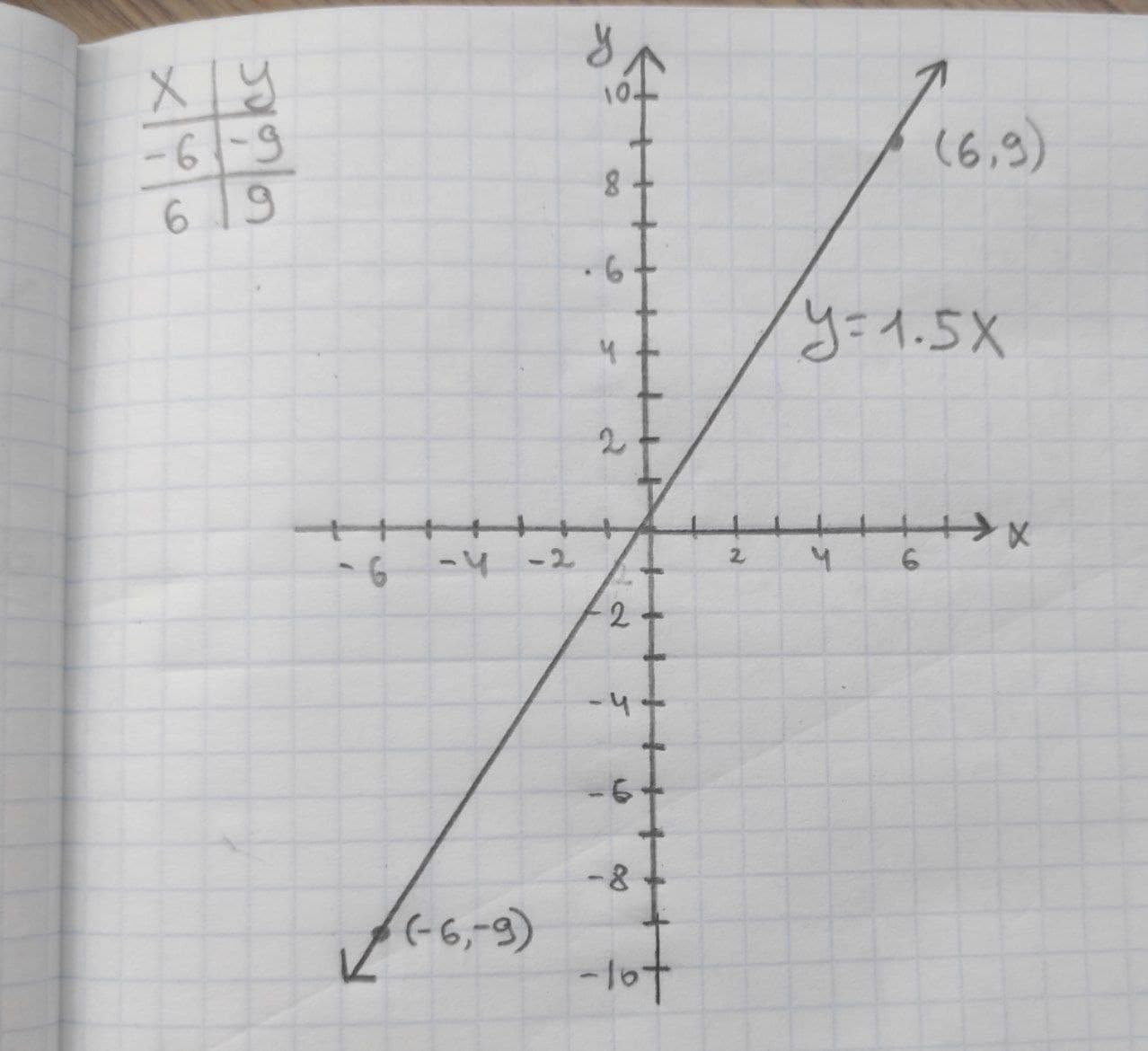Ask question

# Given linear equation y = 1.5x a. find the y-intercept and slope. b. determine whether the line slopes upward, slopes downward, or is horizontal, without graphing the equation. c. use two points to graph the equation.# Given linear equation y = 1.5x a. find the y-intercept and slope. b. determine whether the line slopes upward, slopes downward, or is horizontal, without graphing the equation. c. use two points to graph the equation.

Question
Linear equations and graphsasked 2020-11-29
Given linear equation y = 1.5x
a. find the y-intercept and slope.
b. determine whether the line slopes upward, slopes downward, or is horizontal, without graphing the equation.
c. use two points to graph the equation.

## Answers (1)2020-11-30
The provided linear equation is y = 1.5x. The general form of the linear equation is $$\displaystyle{y}={b}_{{0}}+{b}_{{1}}{x}.$$
1. On comparing the provided linear equation with the general form of the equation, the y-intercept, $$\displaystyle{b}_{{0}}$$ is 0 and the slope, $$\displaystyle{b}_{{1}}$$ is 1.5.
2. The slope of the line is +1.5, so the gradient is +1.5. The slopes upward as the line moves from left to right.
3. Considering the provided equation, the values of y for x = –6 and 6 is obtained as:### Relevant Questionsasked 2020-11-05
Given linear equation y = 0.5x – 2
a. find the y-intercept and slope.
b. determine whether the line slopes upward, slopes downward, or is horizontal, without graphing the equation.
c. use two points to graph the equation.asked 2021-01-19
Given linear equation y = -1+2x
a. find the y-intercept and slope.
b. determine whether the line slopes upward, slopes downward, or is horizontal, without graphing the equation.
c. use two points to graph the equation.asked 2020-11-02
Given linear equation y = 3+4x
a. find the y-intercept and slope.
b. determine whether the line slopes upward, slopes downward, or is horizontal, without graphing the equation.
c. use two points to graph the equation.asked 2020-11-05
Given linear equation y = -0.75x-5
a. find the y-intercept and slope.
b. determine whether the line slopes upward, slopes downward, or is horizontal, without graphing the equation.
c. use two points to graph the equation.asked 2021-01-04
Given linear equation y = 6 − 7x
a. find the y-intercept and slope.
b. determine whether the line slopes upward, slopes downward, or is horizontal, without graphing the equation.
c. use two points to graph the equation.asked 2020-12-21
Given linear equation y = -8-4x
a. find the y-intercept and slope.
b. determine whether the line slopes upward, slopes downward, or is horizontal, without graphing the equation.
c. use two points to graph the equation.asked 2020-12-06
Given linear equation y = -3x
a. find the y-intercept and slope.
b. determine whether the line slopes upward, slopes downward, or is horizontal, without graphing the equation.
c. use two points to graph the equation.asked 2021-02-25
Given linear equation y = -3
a. find the y-intercept and slope.
b. determine whether the line slopes upward, slopes downward, or is horizontal, without graphing the equation.
c.use two points to graph the equationasked 2021-02-12
We give linear equations. For each equation,
a. find the y-intercept and slope.
b. determine whether the line slopes upward, slopes downward, or is horizontal, without graphing the equation.
c. use two points to graph the equation.
y=-3asked 2021-05-14
For the equation (-1,2), $$y= \frac{1}{2}x - 3$$, write an equation in slope intercept form for the line that passes through the given point and is parallel to the graph of the given equation.
...